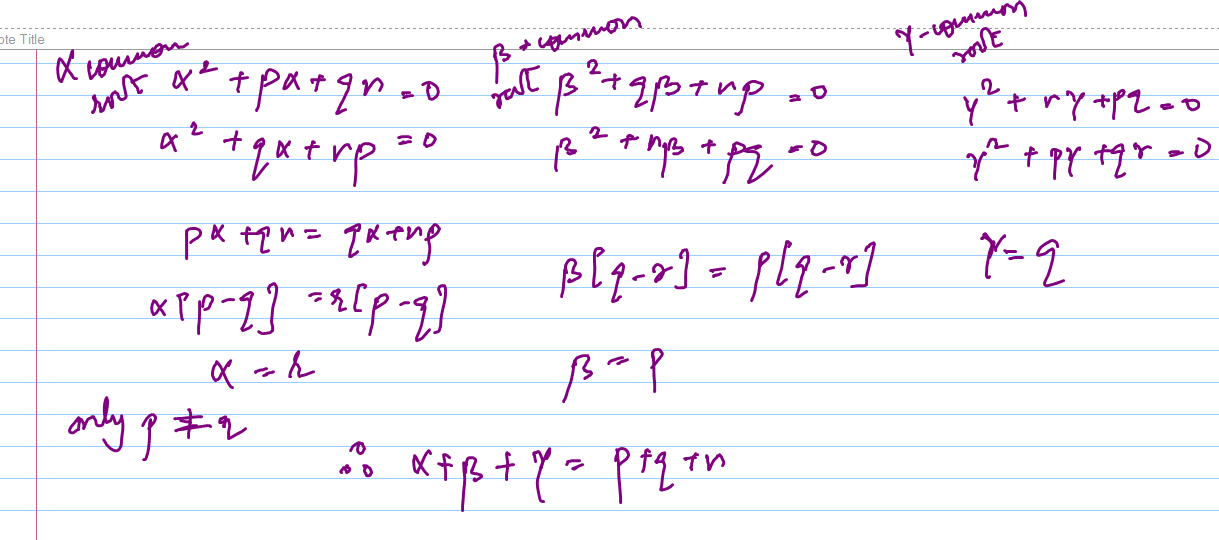×#### Thank you for registering.

One of our academic counsellors will contact you within 1 working day.

Click to Chat

1800-1023-196

+91-120-4616500

CART 0

• 0

MY CART (5)

Use Coupon: CART20 and get 20% off on all online Study Material

ITEM
DETAILS
MRP
DISCOUNT
FINAL PRICE
Total Price: Rs.

There are no items in this cart.
Continue Shopping
```
if every pair of the equation x^2+px+qr=0 , x^2+qx+rp=0 , x^2+rx+pq=0 have a non zero common root then the sum of three common is ?
if every pair of the equation x^2+px+qr=0 , x^2+qx+rp=0 , x^2+rx+pq=0 have a non zero common root then the sum of three common is ?

```
4 years agoSourabh Singh
IIT Patna
2104 Points
``````
4 years ago
```							let a be the common root then satifying the root in all the three eqns. we get,a^2 + ap + qr = 0a^2 + aq + rp = 0a^2 + ar + pq = 0solving all three one by one by elimination method,we geta = r with p = qa = p with q = ra = q with p = rHence, sum of common root => p + q + r                                             => 3p = 3q = 3r respectively.
```
4 years ago
```							Sourabh’s answer can be taken a bit further. Note that we have concluded that r is a root of the 1st quadratic i.e. x2+px+qr = 0. From product of roots, the other root is q. Sum of roots gives q+r = -p, or p+q+r = 0. So, the sum of the common roots is 0
```
4 years ago
Think You Can Provide A Better Answer ?

## Other Related Questions on Algebra

View all Questions »### Course Features

• 731 Video Lectures
• Revision Notes
• Previous Year Papers
• Mind Map
• Study Planner
• NCERT Solutions
• Discussion Forum
• Test paper with Video Solution### Course Features

• 101 Video Lectures
• Revision Notes
• Test paper with Video Solution
• Mind Map
• Study Planner
• NCERT Solutions
• Discussion Forum
• Previous Year Exam Questions